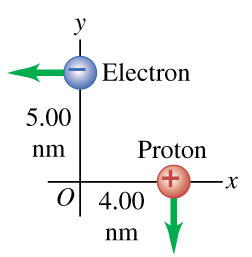# Problem: An electron and a proton are each moving at 795 km/s in perpendicular paths as shown in (Figure 1) .Part AFind the magnitude of the total magnetic force that the electron exerts on the proton.Part  BFind the direction of the total magnetic force that the electron exerts on the proton.

###### FREE Expert Solution

Magnetic force:

$\overline{){{\mathbf{F}}}_{{\mathbf{B}}}{\mathbf{=}}{\mathbf{q}}{\mathbf{v}}{\mathbf{B}}{\mathbf{s}}{\mathbf{i}}{\mathbf{n}}{\mathbf{\theta }}}$

The magnitude of the magnetic field, B:

$\overline{){\mathbf{B}}{\mathbf{=}}\frac{{\mathbf{\mu }}_{\mathbf{0}}\mathbf{qvs}\mathbf{i}\mathbf{n}\mathbf{\theta }}{\mathbf{4}\mathbf{\pi }{\mathbf{r}}^{\mathbf{2}}}}$

95% (183 ratings)###### Problem Details

An electron and a proton are each moving at 795 km/s in perpendicular paths as shown in (Figure 1) .Part A

Find the magnitude of the total magnetic force that the electron exerts on the proton.

Part  B

Find the direction of the total magnetic force that the electron exerts on the proton.